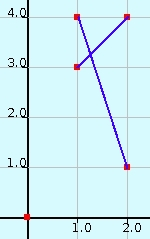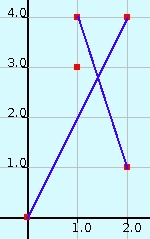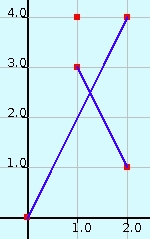### Problem Statement

The prince has just been introduced to geometry in school. The king wants to check his son's progress by giving him the following geometry problem:

You are given the coordinates of N points on the 2-dimensional plane. No two points are coincident and no three are collinear. Find the number of pairs of intersecting line segments whose endpoints are among the given points. The line segments within each pair must not share any common endpoints.

For example, if the given points are {(1, 4), (2, 1), (0, 0), (1, 3) and (2, 4)}, then there are three valid pairs of intersecting line segments as shown in the pictures below.

``````

The king wants you to write a program to solve the above problem so that the answer given by the prince can be checked. The rth point is (xr, yr). The x-coordinates of the points can be generated by using the following recursive definition:

```	x1 = xFirst, and
xi = (xi-1*xProd + xAdd) mod xMod, for 2 ≤ i ≤ N
(Note that (xi-1*xProd + xAdd) may overflow 32-bit integers)
```
The values of yr are defined similarly using yFirst, yAdd, yProd and yMod.

### Definition

 Class: LineSegments Method: intersections Parameters: int, int, int, int, int, int, int, int, int Returns: long Method signature: long intersections(int N, int xFirst, int xAdd, int xProd, int xMod, int yFirst, int yAdd, int yProd, int yMod) (be sure your method is public)

### Constraints

-N will be between 2 and 1200, inclusive.
-xMod and yMod will be between 1 and 1000000, inclusive.
-xFirst, xAdd and xProd will be between 0 and (xMod-1), inclusive.
-yFirst, yAdd and yProd will be between 0 and (yMod-1), inclusive.
-The values of the arguments will be such that among the N generated points (using the above definition), no two points will be coincident and no three will be collinear.

### Examples

0)

 `4` `4` `3` `1` `5` `1` `1` `1` `3`
`Returns: 0`
 The points are (4, 1), (2, 2), (0, 0) and (3, 1).No intersecting line segments.
1)

 `4` `1` `2` `1` `3` `1` `1` `1` `2`
`Returns: 1`
 The points are (1, 1), (0, 0), (2, 1) and (1, 0).Line segment {(0, 0), (2, 1)} intersects with line segment {(1, 1), (1, 0)}.
2)

 `5` `1` `1` `1` `3` `4` `3` `2` `5`
`Returns: 3`
 The example in the problem statement.
3)

 `6` `1` `3` `1` `5` `1` `2` `1` `3`
`Returns: 11`
4)

 `6` `215657` `553897` `915611` `930784` `193666` `323425` `130393` `654599`
`Returns: 15`
 Make sure you take care of overflow.

#### Problem url:

http://www.topcoder.com/stat?c=problem_statement&pm=10949

#### Problem stats url:

http://www.topcoder.com/tc?module=ProblemDetail&rd=14282&pm=10949

keshav_57

#### Testers:

PabloGilberto , ivan_metelsky , zhuzeyuan

Geometry